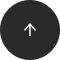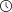- 9 minutes read

# SQL Statistical Analysis Part 3: Measuring Spread of Distribution

Besides knowing the centers of a distribution in your data, you need to know how varied the observations are. In this article, we’ll explain how to find the spread of a distribution in SQL.

Are you dealing with a very uniform or a very spread population? To really understand what the numbers are saying, you must know the answer to this question.

In the second part of this series, we discussed how to calculate centers of distribution. Just like in the case of the center, there are several ways to measure the spread of the distribution in SQL. Also, there are many different definitions for the spread of the distribution. We’ll discuss the most popular ones: the range, the inter-quartile range, the mean absolute, the mean squared deviation, the variance, the standard deviation, and the coefficient of variation. I will also explain the term degrees of freedom. Finally, we’ll consider the difference between variance and standard deviation for samples and for populations. After going through this entry, you’ll be able to find the spread of a distribution in SQL on your own.

# Range

Range is the plain distance between the maximal value and the minimal value that the variable takes. (A variable is an attribute of an observation, represented as a column in a table.) It is the simplest measure of spread. The formula for the range is:

R = vmax – vmin

The T_SQL `MAX` and `MIN` aggregate functions calculate the range of a variable, as shown below:

```USE AdventureWorksDW2014;
SELECT MAX(Age) - MIN(Age) AS Range
FROM dbo.vTargetMail;
```

The code generates the following output:

```Range
-----
70
```

# Inter-Quartile Range

Let’s dive into calculating quartiles in SQL. The median is the value that splits the distribution into two halves. You can split the distribution more—for example, you can split each half into two halves. This creates quartiles: three values that split the distribution into quarters.

Let’s examine this splitting process, the basis for calculating quartiles in SQL. You start with sorting rows (cases, observations) on a selected column (attribute, variable). You define the rank as the absolute position of a row in your sequence of sorted rows. The percentile rank of a value is a relative measure that tells you what percent of all (n) observations have a lower value than a selected value.

By splitting the observations into quarters, you get three percentiles (at 25%, 50%, and 75% of all rows). You can read the values at the quartiles. The first quartile, at the 25% point, is called the lower quartile. The second quartile is the median (50%). The third, at 75%, is the upper quartile. If you subtract the lower quartile (Q1) from the upper quartile (Q3), you get the formula for the inter-quartile range (IQR):

IQR = Q3 – Q1

Calculating quartiles in SQL and getting the IQR is as simple as using the `PERCENTILE_CONT` analytic function:

```SELECT DISTINCT
PERCENTILE_CONT(0.75) WITHIN GROUP (ORDER BY 1.0*Age) OVER () -
PERCENTILE_CONT(0.25) WITHIN GROUP (ORDER BY 1.0*Age) OVER () AS IQR
FROM dbo.vTargetMail;
```

This query returns the following result:

```IQR
---
17
```

Like the median, the IQR is resistant to change. This means it is not sensitive to a wild swing in a single observation. The resistance is logical, because you use only two key observations. When you see a big difference between the range and the inter-quartile range of the same variable, this means that some values in the distribution are quite far away from the mean value.

# Mean Absolute Deviation

For the IQR, you use only two key observations: the lower and the upper quartile. Is there a measure that would take all observations into account? Yes.

You can measure the distance between each value and the mean value and call it the deviation. The sum of all distances gives you a measure of how spread out your population is. But you must consider that some of the distances are positive while others are negative; actually, they mutually cancel themselves out, so the total gives you exactly zero.

The same is true if you were to average the deviations, so this would be a useless measure of spread. You solve this problem by ignoring the positive/negative signs and using the absolute values of the distances between values and the mean.

Calculating the average of the absolute deviations, you get the formula for the mean absolute deviation (MAD):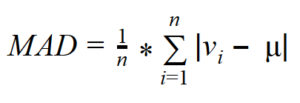From the formula for the MAD, you can see that you need to first calculate the mean. At first, it’s tempting to try this using the AVG aggregate function and using the result as an input in the SUM function. However, SQL Server cannot perform an aggregate function on an expression containing an aggregate or a subquery; therefore, we have to store the mean value (from `AVG`) in a variable:

```DECLARE @mean AS NUMERIC(10,2);
SET @mean = (SELECT AVG(1.0*YearlyIncome) FROM dbo.vTargetMail);
SELECT SUM(ABS(YearlyIncome - @mean))/COUNT(*) AS MAD
FROM dbo.vTargetMail;
```

You get the following output:

```MAD
------------
25474.966405
```

# Mean Squared Deviation

Another way of avoiding the problems of deviation signs to square each deviation. With a slight modification of the MAD formula — specifically, calculating the average of the squared deviations instead of the absolute deviations — you get the formula for the mean squared deviation (MSD):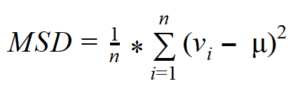You might have asked yourself why I did not use any window aggregate functions for the MAD calculation. Of course, this is possible. I will do it for the MSD calculation. Let’s try with the following query!

```SELECT
SUM(
SQUARE(YearlyIncome -
(AVG(1.0*YearlyIncome) OVER())
)
) / COUNT(*) AS MSD
FROM dbo.vTargetMail;
```

Unfortunately, this approach is quite naive. The query returns `error 4109 - windowed functions cannot be used in the context of another windowed function or aggregate`.

You need to use the window aggregate function inside a common table expression, and then do the final aggregation in an outer query. The following code snippet shows how it’s done:

```WITH MSDCTE AS
(
SELECT
YearlyIncome,
AVG(1.0*YearlyIncome) OVER() AS Deviation
FROM dbo.vTargetMail
)
SELECT SUM(SQUARE(YearlyIncome - Deviation)) / COUNT(*) AS MSD
FROM MSDCTE;
```

This returns the desired result:

```MSD
----------------
1042319181.07085
```

# Degrees of Freedom and Variance

Let’s suppose that you have only one observation (n=1). This observation is also your sample mean, but there is no spread at all. You can calculate the spread only if n exceeds 1. Only the (n–1) pieces of information help you calculate the spread, considering that the first observation is your mean. These pieces of information are called degrees of freedom.

Think of degrees of freedom as the number of pieces of information that can vary. For example, imagine a variable that can take five different discrete states. You only need to calculate the frequencies of four states to know the distribution of the variable; the frequency of the last state is determined by the frequencies of the first four states calculated. They cannot vary because the cumulative percentage of all states must equal 100.

The sum of all deviations, without ignoring positive/negative signs, is always zero. Because of that, the formula for variance uses squared deviations. There are only (n–1) deviations free; the last one is strictly determined by the others. The definition of Variance (Var) is similar to the definition of the MSD; you just replace the number of cases n with the degrees of freedom (n–1):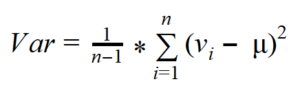This is the formula for the variance of a sample, which can be used as an estimator for the variance of the population. Now imagine that your data represents the complete population. In that case, all the observations contribute to the variance calculation equally, and the degrees of freedom make no sense. The variance of a population (VarP) is defined, then, with the same formula as the MSD: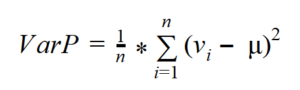Of course, when you have a large sample, the difference between Var and VarP is minimal.

Transact-SQL includes an aggregate function (the VAR function) that calculates the variance of a sample as an estimator. The VARP function calculates the variance of the population. Using either in a query is very simple. The following example calculates both variances for the “`YearlyIncome`” column. It also compares them in two ways: by dividing them, and by dividing the number of cases minus one by the number of cases. This last shows that the difference is only a result of the degrees of freedom used in calculating the sample’s variance as an estimator for the population variance:

```SELECT VAR(1.0*YearlyIncome) AS SampleVariance,
VARP(1.0*YearlyIncome) AS PopulationVariance,
VARP(1.0*YearlyIncome) / VAR(1.0*YearlyIncome) AS SampleVsPopulation1,
(1.0 * COUNT(*) - 1) / COUNT(*) AS SampleVsPopulation2
FROM dbo.vTargetMail;
```

The query returns the following result:

```SampleVariance   PopulationVariance SampleVsPopulation1 SampleVsPopulation2
---------------- ------------------ ------------------- -------------------
1042375574.46912 1042319181.07081   0.999945899156027   0.999945899156
```

# Standard Deviation and Coefficient of Variation

To compensate for having the deviations squared in the formula for the variance, you can take the square root of the variance. This is the definition of the standard deviation (σ):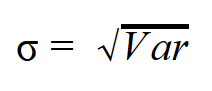You can use this formula to calculate standard deviation in SQL, both of the population and of a sample – just use the appropriate variance in the formula.

Suppose we derived the absolute measures of a spread. The interpretation is quite evident for a single variable: the bigger the values of the measures are, the more spread out the variable in the observations is. But absolute measures cannot be used to compare the spread between two or more variables. Therefore, we need to derive relative measures.

We can derive the relative measures of the spread for any of the absolute measures mentioned. However, we will only do the most popular one: the standard deviation. The definition of the relative standard deviation (also known as the coefficient of variation, or CV) is a simple division of the standard deviation by the mean value: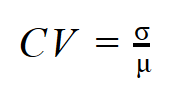T-SQL includes an aggregate function to calculate the standard deviation in SQL for the population (STDEVP) and one to calculate the standard deviation for a sample as an estimator (STDEV). Calculating the standard deviation in SQL as well as the coefficient of the variation, therefore, is simple. The following query calculates standard deviations for the “Age” and “YearlyIncome” columns and the coefficient of variation for them:

```SELECT STDEV(1.0*Age) AS StDevAge,
STDEV(1.0*YearlyIncome) AS StDevIncome,
STDEV(1.0*Age) / AVG(1.0*Age) AS CVAge,
STDEV(1.0*YearlyIncome) / AVG(1.0*YearlyIncome) AS CVIncome
FROM dbo.vTargetMail;
```

Here is the result:

```StDevAge          StDevIncome       CVAge              CVIncome
----------------  ----------------  -----------------  -----------------
11.5178146121881  32285.8417029682  0.241654328044298	  0.563395923529214
```

After calculating the standard deviation in SQL you can see that the standard deviation for “YearlyIncome” is much higher than for “Age”; however, the relative spread, the coefficient of variation, is not that different.

# Conclusion

Centers of distribution, especially the mean value, are probably the most abused measures in statistics. Mean does not mean much without mentioning the spread. There are several measures of spread: standard deviation, variance, and the coefficient of variation are the most important ones. As now you know more about measuring the spread of a distribution in SQL and topics such as calculating quartiles in SQL or writing queries to obtain standard deviation in SQL are not foreign to you, you’ve taken your statistical analysis to the next level!